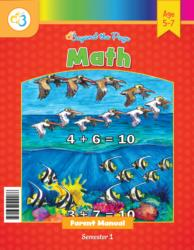# Common Core Alignment

CCSS.Math.Content.1.OA.1 - Use addition and subtraction within 20 to solve word problems involving situations of adding to, taking from, putting together, taking apart, and comparing, with unknowns in all positions, e.g., by using objects, drawings, and equations with a symbol for the unknown number to represent the problem.2

## 5: MathUnit 3: Addition and Subtraction to 10
Lesson 3: Getting on Board with Addition
Lesson 4: More Practice with Addition
Lesson 5: Finding Differences
Lesson 6: Adding and Subtracting to Solve Problems
Final Project: My Sticker BookUnit 5: Addition and Subtraction to 20
Lesson 7: Problem Solving